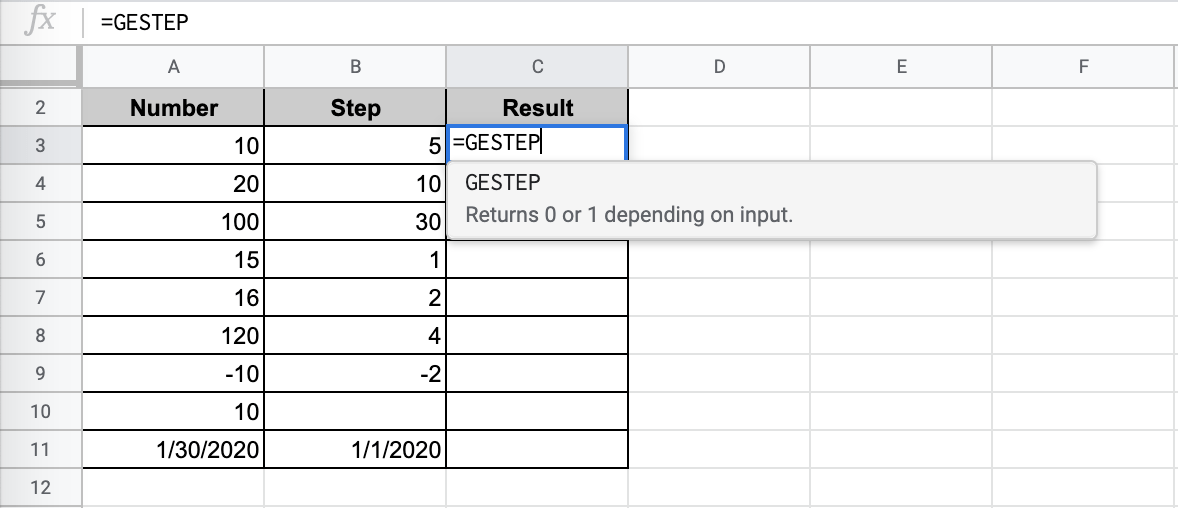# How to Use GESTEP Function in Google Sheets

The `GESTEP` function in Google Sheets is useful for Engineering purposes, as it determines a rate is strictly greater than or equal to the provided step value.

The `GESTEP` function is a rather simple function, used specifically for engineering purposes in Google Sheets.

The function returns the value of 1 if the rate is strictly greater than or equal to the provided step value, and the value of 0 otherwise. If there is no step value that is provided in the example, then the default value of 0 is used.

Let’s take an example.

Say that we are required to know if a given value is great than another provided step value. It is an easy comparison function, especially for engineering purposes.

So how should we approach this?

Simple – we use the `GESTEP` function.

## The Anatomy of the GESTEP function

The syntax (the way we write) the `GESTEP` function is simple:

`=GESTEP(value,[step])`

Let’s break this down to understand the syntax of the `GESTEP` function and each term meaning:

• `=` the equal sign is how we begin any function in Google Sheets
• `GESTEP` is our function. We need to add the value attribute for it to work properly.
• `value` is the attribute of this function. It is the input number which will be used in the comparison function.
• `step` is an optional attribute.

## A Real Example of Using GESTEP Function

Let’s look at some examples below to see how to use the `GESTEP` function in Google Sheets.

### Determining the Rate Greater Than or Equal to The Step Value in Google Sheets

This is a simple problem that will illustrate how you can use `GESTEP` in your spreadsheets and formulas, especially for engineering applications.This is a flexible function as you can input the necessary factors in a number of ways. This function accepts direct number values or a simple cell reference without any quotes.

The function with  a direct number value is as follows:

`=GESTEP(10,5)`

And the function with a cell reference is:

`=GESTEP(B3,C3)`

As a result, we get 1 or true, determining that the rate is greater than the step inputted.

Isn’t it quite simple?

This simple problem can be practiced. Use the link below to use our spreadsheet sample:

## How to Use GESTEP Function in Google Sheets

In this section, we will show you a step-by-step process on how to use the `GESTEP` function in Google Sheets.

1. To begin, click on the cell where you want to show your result to make it an active cell. For this guide, we will use the cell C3. Add the ‘=‘ sign to start our function.1. Type in `GESTEP`.1. You will notice it will give us further information about using the function.1. Choose the value you want to compare to the step.1. Optional: Choose the step you are comparing the value with. If you do not choose a step, you will automatically choose the value 0.1. Close the function with another bracket ‘)‘. C3 should display the result.1. Copy the formula for the rest of the values you want to compare.There you have it! You are now able to use the `GESTEP` function in Google Sheets as you wish. Now that you have a grasp on how to combine functions in your spreadsheets, you can combine this with other Google Sheets formulas to make really powerful data documents!Our goal this year is to create lots of rich, bite-sized tutorials for Google Sheets users like you. If you liked this one, you'll love what we are working on! Readers receive ✨ early access ✨ to new content. There will be no spam and you can unsubscribe at any time.

##### You May Also Like## How to Use SUMIFS Function in Google Sheets

The SUMIFS function in Google Sheets is useful if you want to get the sum of cells that…## How to Create Dot Plots in Google Sheets

Learning how to create dot plots in Google Sheets is useful for creating a simple and easy-to-understand representation…## How to Use ISNUMBER Function in Google Sheets

The ISNUMBER function in Google Sheets is used to determine if a value is a number. This function…## How to Use RANK.AVG Function in Google Sheets

The RANK.AVG function in Google Sheets is used to determine the rank of a specific value in a…